# Future Indoor Global Positioning System (GPS) Using White LEDs?

by Jiun Bin Choong, Department of Electrical and Computing Systems, Monash University

## Abstract

White light-emitting diodes (LEDs) are fast replacing conventional lighting because of their excellent energy efficiency, which makes them a more sustainable source of lighting. Unlike conventional light sources, white LEDs designed for illumination can also transmit high speed signals, thus unlocking an unprecedented opportunity to create a completely new positioning system. The new systems will be complementary to the familiar Global Positioning System (GPS) as they will be more accurate and will be usable indoors. Installing the new systems will be almost as 'simple as changing a light bulb'. Applications which could be based on these new systems extend from asset tracking, to guidance system for robots, to navigational aids for the visually impaired. In this project white LEDs are replaced with coloured LEDs for simplicity and a small scale model representing a typical room environment has been built. Preliminary results have shown that the ceiling mounted coloured LEDs can be used to estimate positions of the receiver accurately (with errors less than one centimetre) through the concept of photogrammetry.

Keywords: Light, LEDs, Photogrammetry, Colour, Coordinates, Pixel

## Introduction

White light-emitting diodes (LEDs) designed for illumination can also be modulated up to a few megahertz to transmit their coordinate information to a portable receiver (Grubor et al., 2008). This makes the new emerging technology of visible light communication a very suitable candidate for developing indoor positioning systems. In future the white LEDs will replace incandescent and fluorescent lighting due to their higher energy efficiency, longer life expectancy, higher tolerance to humidity and environmental friendliness (Swook et al., 2010). More importantly, indoor positioning system introduces an enormous range of applications including asset tracking, providing navigational aids to visually impaired, guidance system for mobile robots and industrial automation.

The performance of indoor positioning systems based on radio technology is limited by the inevitable issues of multipath distortions in indoor signal transmissions (Hui et al., 2007). On the other hand, it has been suggested that indoor positioning using visible light can achieve accuracy in the order of micrometres and decimetres (Mautz and Tilch, 2011).

Moreover, visible light transmissions are confined to the room in which they originate since light cannot pass through walls or other opaque barriers, therefore it is '[easy to] secure transmissions against eavesdropping, and prevents signal interference between rooms' (Kahn and Barry, 1997: 1). The main source of noise for visible light communication systems is the intense ambient noise in many indoor environments, arising from the sunlight and other light emitting sources like incandescent lightings which could potentially degrade the performance of the indoor positioning system.

Recent research on indoor positioning using white LEDs has proposed a number of techniques including time of arrival (TOA), time difference of arrival (TDOA), angle of arrival (AOA), and received signal strength (RSS) (Mao et al., 2009). However, due to the restricted distance between the transmitter and the receiver indoors, TOA and TDOA measurement techniques may not be feasible for indoor analysis since the time difference between light rays reaching the receiver is only a few nanoseconds. Localising (position estimation) by using RSS also has some limitations since there will be some variations between the brightness of the LEDs. A promising technique for indoor positioning through visible light is AOA, which measures the angle of the light ray incident onto the receiver.

In this work, a hemispherical lens is attached to the receiver, enlarging the receiver's field of view and allowing it to receive more signals under most circumstances (Wang et al., 2013). The receiver can estimate its position more accurately with more coordinate information from additional received signals.

The localisation technique described in this article is based on photogrammetry which considers a single reference point on the photographic image used to estimate the position of the receiver (Uchiyama et al., 2008). However, the addition of hemispherical lens at the receiver complicates the geometrical analysis of the image since the light ray is refracted twice, once at each surface of the lens. The system has been analysed using Fresnel's equations (Pedrotti et al., 2007).

In summary, this article illustrates that the concept of visible light communication can be used to develop indoor positioning system. The System Description section explains the details of the experimental setup, assumptions involved in the experiment and limitations to the photogrammetry technique. The Methodology section describes the indoor localisation technique based on photogrammetry (Uchiyama et al., 2008), where the position of the receiver can be estimated by analysing the reference point on the captured image. Preliminary results illustrate that ceiling-mounted LEDs can be used for indoor positioning with accuracy in the order of millimetres, similar to what Mautz and Tilch (Mautz and Tilch, 2011) have suggested. Finally, further studies for this research are to use 3D triangulation rather than simple geometry to localise and updating the experimental setup with modulating white LEDs for transmitting position information (Kahn and Barry, 1997).

## Literature Review

Visible light communication technology can be used to develop indoor positioning system since white LEDs, designed for illumination, can also be modulated rapidly to transmit their position information to a receiver (Grubor et al., 2008). The major benefit of using visible light communication technology is that visible light transmissions are secure 'against eavesdropping and prevents signal interference between rooms' (Kahn and Barry, 1997: 1). Similar to the work of Uchiyama et al. (2008), this research will use only a single reference point on the captured photographic image to estimate the position of the receiver according to the photogrammetry technique. Further studies for this work are to use at least three reference points on the image to perform a 3D triangulation analysis to localise the receiver. Photogrammetry is a measurement technique for determining the position of the photographed object (Hallert, 1960). In this research, the photographed object is the image of each LED and the single reference point is based on the position of the brightest ray (brightest pixel) incident onto the receiver. Moreover, Rahman et al. (Rahman et al., 2011) demonstrated that more pixels in the image can improve the accuracy of position estimation. Therefore, it is expected that a higher resolution (more pixels) image should estimate a more accurate position of the receiver.

The addition of the hemispherical lens helps to expand the receiver's field of view, allowing it to receive more signals under most circumstances (Wang et al., 2013). However, the geometrical analysis of the image becomes complicated since light will be refracting twice during its path to the receiver. Therefore, it is necessary to consider only the brightest ray since Fresnel's equations indicate that the brightest ray will only refract once through the flat surface of the hemispherical lens (Pedrotti et al., 2007). This simplifies the geometrical analysis of the image as the incidence angle (AOA) of the brightest ray can be easily calculated through the fundamental Snell's Law of refraction. Consequently, the position of the receiver relative to the position of the LEDs inside the model can be computed.

## System Description

This article presents a system where a receiver is able to localise inside a small-scale model of a room. Figure 1 shows the experimented system which has been built. The experimental setup has three coloured LEDs mounted on the ceiling and a receiver on the floor. The receiver has a hemispherical lens used for a wider field of view, a translucent screen with a marked grid for photogrammetry analysis, and a camera used for capturing images of the LEDs and the grid.

The dimensions of the model are approximately 0.5m x 0.5m x 0.5m. A linear X-Y coordinate system is drawn on the flooring to show the exact location of the receiver inside the model. This is used to calculate the error between the actual and estimated position of the receiver.

The model described in this article uses a simple system with red, green and blue (RGB) LEDs rather than white LEDs. It is also assumed that the receiver knows the position of each colour LED. In future systems white LEDs which use modulated light to transmit their coordinates will be used. A further simplification in this experiment is that it is assumed that the position of the receiver lies within a plane at a known distance from the ceiling. This means that position information can be calculated from the image of a single LED. In later experiments this will be extended to 3D triangulation using three LEDs.

The colour of the image of each LED is identified using the mathematical program MATLAB. The program separates the captured coloured image into red, green and blue (RGB) components and from the proportions of each colour, the colour of each LED can be determined.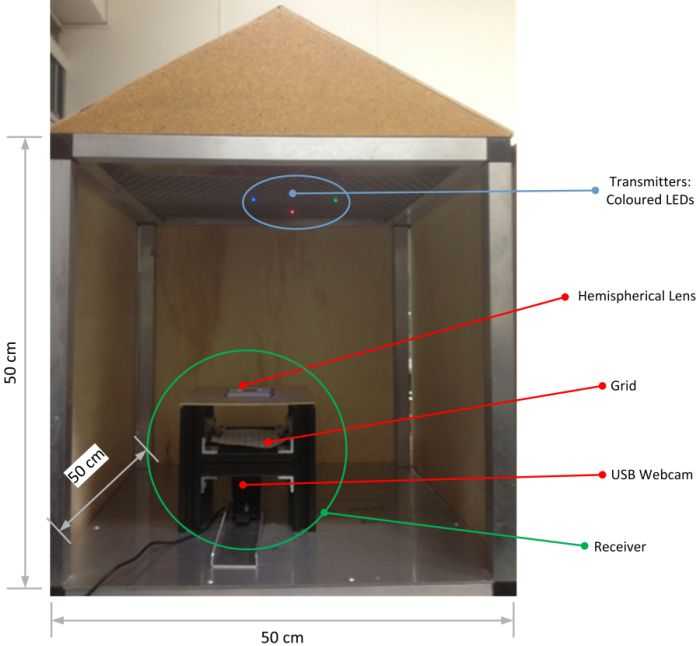Figure 1: Photo of Experimental Setup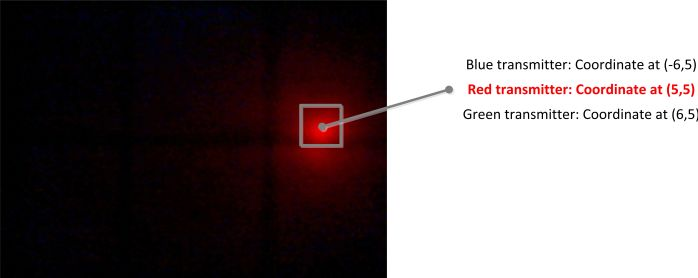Figure 2: Captured image of red light

At the start of the experiment, the receiver is placed on the floor of the model at a position where it can see at least one LED. Next the USB camera captures an image of the LEDs and the grid. This is then processed using MATLAB. The localising technique calculates the position of the receiver relative to the LEDs. This is achieved by first identifying the image of each LED within the picture. Then the brightest pixel within each LED image is found and from this using geometry, the position of the receiver relative to the LEDs is calculated.

For the case of different coloured LEDs, each LED is identified by its colour. This is achieved in MATLAB by separating the image into red, green and blue (RGB) colour components. Each colour LED has characteristic proportions of RGB. Figure 3 shows experimental results for the red LED. The figure shows how the intensity varies across one scan line of the picture. The red component has a clear peak, while the blue and green components have low intensity levels across the scan.

In this program the position of the brightest pixel is used to identify the position of the LED within the picture. In practice a number of factors may limit the accuracy of this approach. One problem is saturation of the image when the received light is too bright for the camera. This is shown in Figure 4. This technique is also limited by the number of pixels in the image. Finally each LED is not a point source, and the size of the image depends on the size of the LED.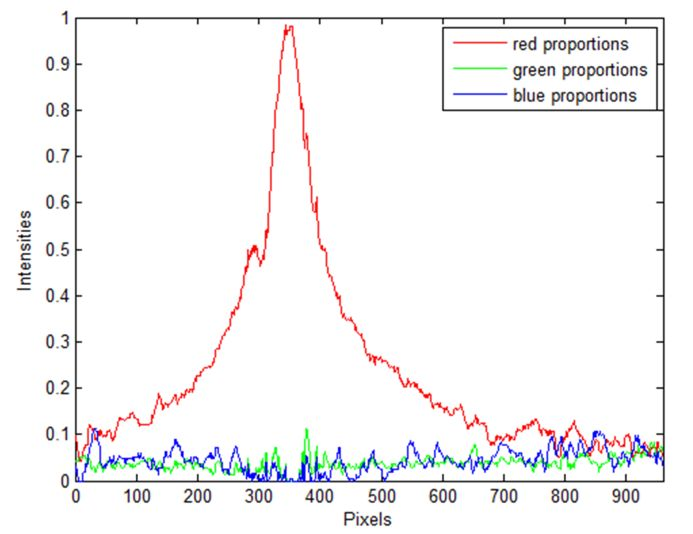Figure 3: Determining the colour of image - example of red light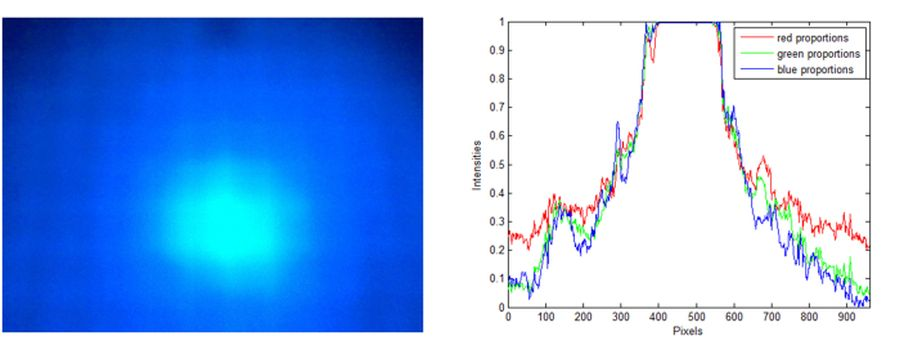Figure 4: figure (left) shows actual photo of saturated image; figure (right) shows multiple maximum intensities from saturated image

## Methodology

The localisation technique described in this article is based on the concept of photogrammetry (Appendix A). It requires one reference point on the photographic image used for estimating the receiver's position. Once the reference point has been identified, AOA technique can be used to re-trace the light ray's propagation path back to the position of the transmitter (coloured LEDs). Consequently, the receiver can successfully localise relative to the transmitter's position inside the model.

The calculations use the position of the brightest pixel within the image, given in a coordinate system based on the image dimension. This is shown as the 'local coordinate system' in Figure 5. This is used to calculate the position of the receiver within the room. This position is given using a 'global coordinate system' which is defined relative to the walls and floor of the room. See Figure 5.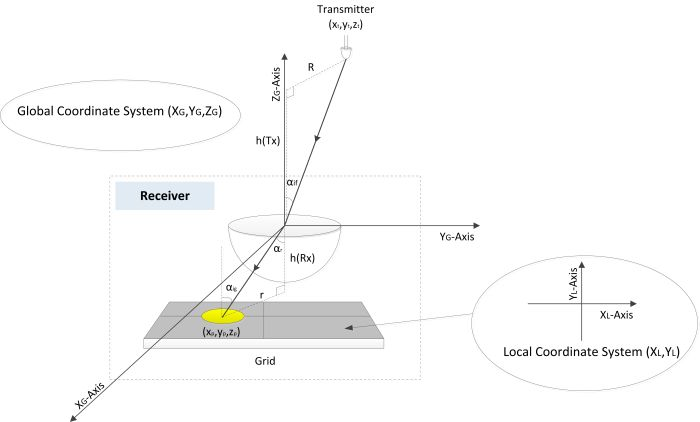Figure 5: Local and Global Coordinate Systems

Once the local coordinate of the brightest pixel ($x_p,y_p$) has been identified in MATLAB, the angle of arrival (AOA) of the brightest ray incident onto the grid can be estimated through the trigonometric tangent equation:$r=\sqrt{x^2_p+y^2_p}$$a_{ig}=\tan^{-1}\bigg(\frac{r}{h(Rx)}\bigg)$

Since light will be refracting twice through the hemispherical lens, it becomes difficult to measure the second point of angle of refraction where the light ray exits the hemispherical lens (Figure 6). See Appendix B for more information.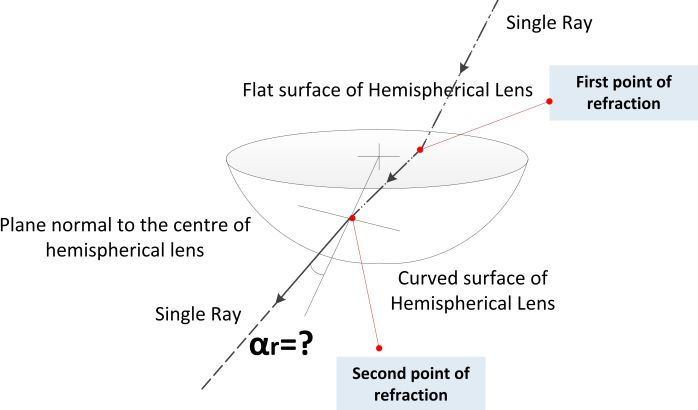Figure 6: Finding the angle of refraction α_r from the curved surface

Fresnel's equations (Appendix C) help to simplify the geometry of light ray path as the ray (s- and p-polarised) overall reflects at minimum when the incident angle (θi) onto the glass is at zero degrees (Figure 7).

Fresnel's Equations:$Rs=\Big|\frac{n_{glass}\cos\theta_i-n_{air}\cos\theta_t}{n_{glass}\cos\theta_i+n_{air}\cos\theta_t}\Big|^2$$Rp=\Big|\frac{n_{glass}\cos\theta_t-n_{air}\cos\theta_i}{n_{glass}\cos\theta_t+n_{air}\cos\theta_i}\Big|^2$Figure 7: Fresnel's equations s- and p-polarised reflection coefficients

Hence, the light ray with the lowest optical power reflections (measured by s- and p- polarised reflection coefficients) when travelling inside the hemispherical lens has the incident angle of zero onto the curved surface of the lens. Therefore the light ray with the lowest reflection (brightest ray) in fact only refracts once through the flat surface of the lens and passes through the curved surface of the lens without second refraction (Figure 8).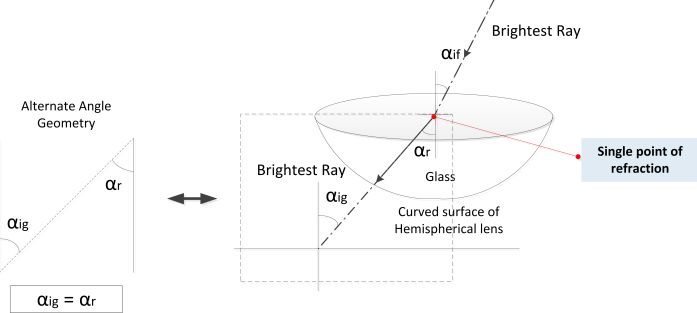Figure 8: Simplified geometrical analysis of light ray

The angle of refraction$\alpha_r$ off the flat surface of the hemispherical lens equals to the angle of incident ray$\alpha_{ig}$ onto the grid due to alternate angle geometry. Next, the incident angle$\alpha_{if}$ of the brightest ray onto the flat surface of the hemispherical lens can be calculated through the fundamental Snell's law of refraction (Figure 8):$\alpha_{if}=\sin^{-1}\Big(\frac{n_{glass}}{n_{air}}*\sin(\alpha_r)\Big)$

Assuming h(Tx) (height between the flat surface of the hemispherical lens and the ceiling) is known, R (the distance between the receiver and the transmitter) can be estimated through trigonometric tangent function (Figure 5):$R=h(Tx)*\tan(\alpha_{if})$

Finally, since light travels in straight line, the coordinate of the receiver$(x_{estimate},y_{estimate})$ can be estimated by first finding the angle θ from the local coordinate system based on the position of the brightest pixel. The angle θ is consistent in both local and global coordinate systems due to alternate angle geometry (Figure 9):$\theta=\tan^{-1}\Big(\frac{x_p}{y_p}\Big)$$x_{estimate}=R*\sin\theta+x_t$$y_{estimate}=R*\cos\theta+y_t$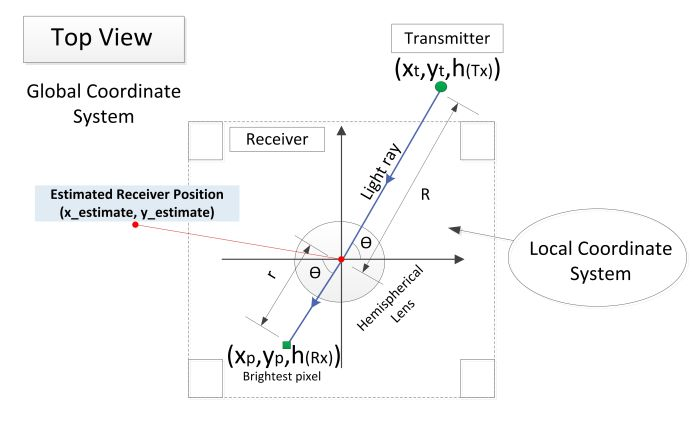Figure 9: Top view of the overall system

## Preliminary Results and Discussions

The aim of this research was to examine whether, through the use of visible light, the receiver could successfully localise inside the model with minimal error when comparing to the actual position of the receiver.

One possible cause of error between measured and calculated position of the receiver is the misalignment of the USB camera when placed under the receiver. To observe the effect of the misalignment, several trials were performed where the receiver was placed in three separate positions to receive the three different coloured LED lights inside the model (Figure 10). Next, the USB camera captured the same image up to five times (trials) with small adjustments to the camera's position each time before the photo was taken. In Figure 11 and Figure 12, each colour trial denotes the error from estimating the position of receiver according to that particular colour light. The average colour denotes the average errors from the five trials.Figure 10: Position to target green light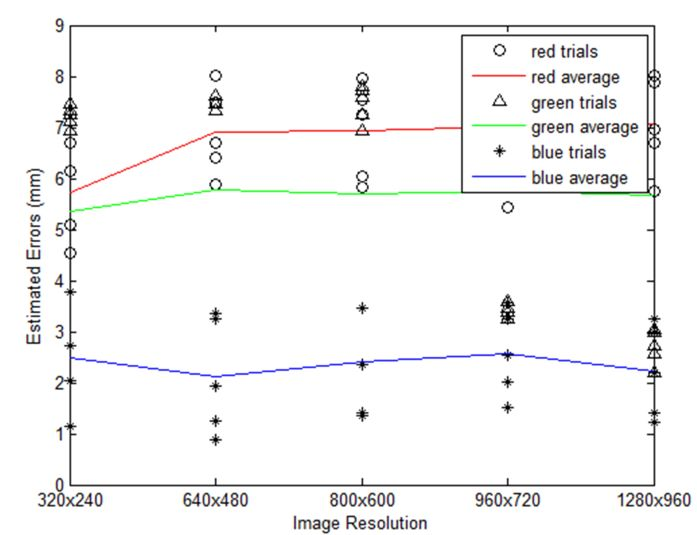Figure 11: Errors along x-axis

 Table of Results Trial1 Trial2 Trial3 Trial4 Trial5 Average Image Resolution Error(mm) Error(mm) Error(mm) Error(mm) Error(mm) Error(mm) 320x240 6.1533 6.6882 5.0805 6.1515 4.5460 5.7239 640x480 6.6882 8.0248 6.4196 7.4882 5.8848 6.9011 800x600 7.5438 7.9711 6.0443 7.2383 5.8308 6.9257 960x720 7.4014 7.9358 6.3293 7.9341 5.4378 7.0077 1280x960 6.9557 8.0246 6.6866 7.8905 5.7504 7.0616

Table 1: Example of Errors along x-axis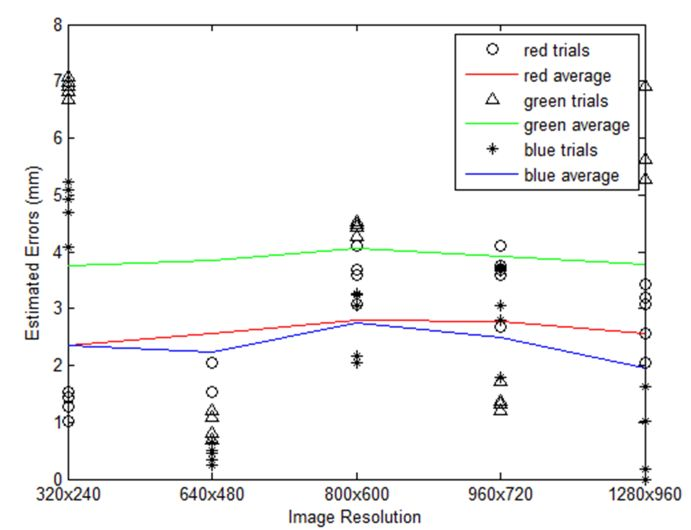Figure 12: Errors along y-axis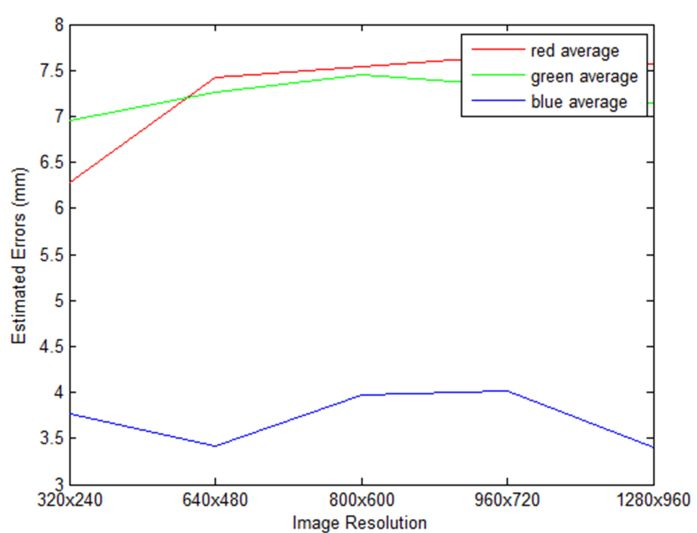Figure 13: Root Mean Squared Error Distance (RMSED)

The root mean squared error distance (RMSED) measures the absolute error distance between the estimated and actual position of the receiver:$RMSED=\sqrt{(x_{actual}-x_{estimate})^2+(y_{actual}-y_{estimate})^2}$

The large variations between the trial and average errors from Figure 11 and Figure 12 highlight the concern that slight misalignment of the USB camera from the receiver's centre does result in large differences between measurements. Furthermore, according to Figure 13, the calculations involving all three individual reference points from each coloured LEDs have RMSED values below 1 cm. This illustrates that the error between the actual and estimated position of the receiver is marginally small which is similar to the conclusion drawn from Mautz and Tilch (Mautz and Tilch, 2011) survey. Interestingly, all error plots (Figures 11, 12 and 13) indicate that there are little differences in errors between images of higher resolutions and of lower resolutions when estimating the receiver's position. This outcome in fact conflicts with Rahman, Haque et al. (Rahman, Haque et al., 2011) own findings since higher resolution images do not necessarily calculate a more accurate position of the receiver.

However, other factors such as refractions at LEDs, colour dispersions at the hemispherical lens and small misalignment between hemispherical lens and the grid may also help to explain the variations between the estimated and actual position of the receiver (Appendix D). Nevertheless, the preliminary results have empirically shown that developing indoor positioning system through the concept of visible light communication can achieve great accuracy, just as Mautz and Tilch (Mautz and Tilch, 2011) have suggested.Figure 14: Overview of Estimate and Actual Positions of Receiver inside model

## Conclusion and Further Studies

This article proposed that indoor positioning system can be developed through white LEDs based on the concept of visible light communication and photogrammetry (Uchiyama et al., 2008). In this work, white LEDs are replaced with coloured LEDs for simplicity and several trials of camera positioning at the receiver were tested. Just as Mautz and Tilch (2011) suggested in their survey of optical indoor positioning systems, preliminary results in this work have shown that positioning using visible light can achieve great accuracy (in the order of millimetres). The errors in the position estimation of the receiver can be minimised if factors such as refractions at LEDs, colour dispersions at the hemispherical lens and small misalignment between hemispherical lens and the grid were also considered. Limitations on the photogrammetry technique include saturation of the image when the received light is too bright for the camera, number of pixels in the image, LED is not a point source and the size of image depends on the size of LED. Limitations on the experimental setup include positioning of the receiver restricted to the model's size and that white LEDs were replaced with coloured LEDs with the assumption that position information is known to the receiver.

It is recommended that further research be carried out to examine 3D triangulation technique rather than simple geometrical analysis since some assumptions may be impractical, such as the height between receiver and transmitter may be inconsistent due to the irregular structure of the ceiling in some indoor scenarios. In addition, the proposed system could be further developed using white LEDs for wireless data transmission through intensity modulation and direct detection (IM/DD) technique (Kahn and Barry, 1997).

## Acknowledgements

Special thanks to Professor Jean Armstrong for her time and guidance; Dr Ahmet Sekercioglu, Dr Tom Q. Wang and Sarangi Dissanayake for their advice; James Yew for his assistance with experimental setup; Summer Research Programme at Monash University for 12 weeks of extensive research training.

Many thanks to my family and friends, the 'MBs' for their continuous support.

## List of Figures

Figure 1: Photo of Experimental Setup (photo: author's own)

Figure 2: Captured image of red light (photo: author's own)

Figure 3: Determining the colour of image - example of red light

Figure 4: Figure (left) shows actual photo of focused image (photo: author's own); figure (right) shows multiple maximum colour intensities from focused image

Figure 5: Local and Global Coordinate Systems

Figure 6: Finding the angle of refraction α_r from the curved surface

Figure 7: Fresnel's equations s- and p-polarised reflection coefficients

Figure 8: Simplified geometrical analysis of light ray

Figure 9: Top view of the overall system

Figure 10: Position to target green light (photo: author's own)

Figure 11: Errors along x-axis

Figure 12: Errors along y-axis

Figure 13: Root Mean Squared Error Distance (RMSED)

Figure 14: Overview of Estimate and Actual Positions of Receiver inside model

Figure 15: Vertical Photogrammetry over a terrain

Figure 16: Cross section of light ray passing through flat surface of hemispherical lens

Figure 17: Cross section of light ray passing through curved surface of hemispherical lens

Figure 18: Visual Illustration of TE and TM waves

Figure 19: Additional issue: Light rays refracting at LED

Figure 20: Additional issue: Colour Dispersion

Figure 21: Additional issue: Misalignment between grid and lens

## List of tables

Table 1: Examples of Errors along x-axis

## Appendices

##### Appendix A: Photogrammetry

Photogrammetry is a method of obtaining measurements through photography in order to determine geometric characteristics, such as position of the photographed object (Hallert B. 1960). Photogrammetry started around 1850, where aerial photogrammetry was used for mapping purposes (Figure 15).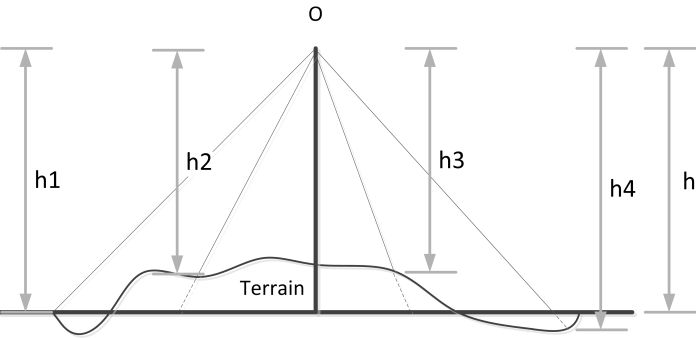Figure 15: Vertical Photography over a terrain

##### Appendix B: Further Explanation Regarding Calculation Complications

Fundamental Snell's law of refraction:$n_{air}*\sin(\alpha_i)=n_{glass}*\sin(\alpha_r)\qquad{Figure 16}$$n_{glass}*\sin(\alpha_i)=n_{air}*\sin(\alpha_r)\qquad{Figure 17}$

where nair and nglass refers to the refractive indices for air and glass medium respectively.Figure 16: Cross section of light ray passing through flat surface of hemispherical lens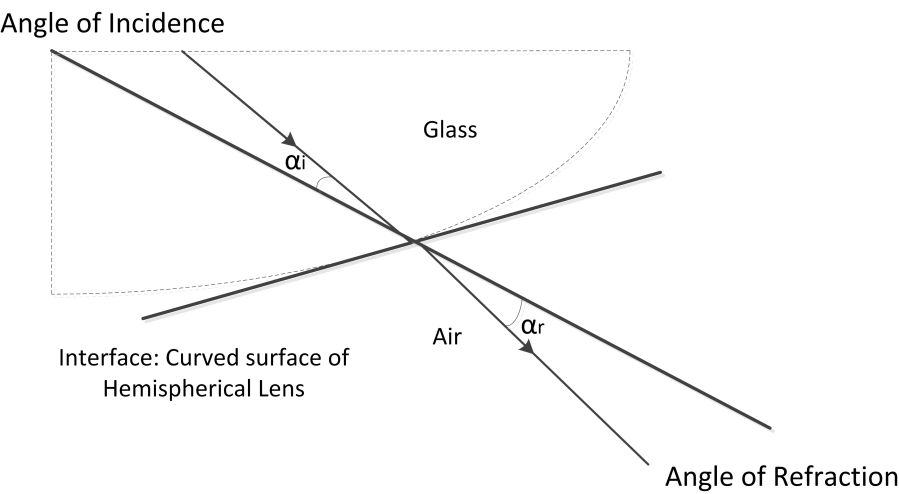Figure 17: Cross section of light ray passing through curved surface of hemispherical lens

Complications arise because the interface between the two mediums is no longer perfectly horizontal or vertical (Figure 17). It is more difficult to determine the angle of refraction$\alpha_r$ of the light ray when the interface is curved compared with the flat interface.

##### Appendix C: Fresnel's Equations

Fresnel's equations describe the behaviour of light when moving from one medium to another. More specifically, it describes the 'fraction of incident energy transmitted or reflected at a plane surface' (Pedrotti L. F. et al., 2007). A plane can be described as a flat two dimensional surface, and in this work the plane denotes the interface between the air and glass medium where the light ray interacts.

Fresnel's Equations:$Rs=\Big|\frac{n_{glass}\cos\theta_i-n_{air}\cos\theta_t}{n_{glass}\cos\theta_i+n_{air}\cos\theta_t}\Big|^2$$Rp=\Big|\frac{n_{glass}\cos\theta_t-n_{air}\cos\theta_i}{n_{glass}\cos\theta_t+n_{air}\cos\theta_i}\Big|^2$

In Fresnel's equations, Rs and Rp are alternative representations for transverse electric field (TE) and transverse magnetic (TM) field reflection coefficients since light is considered as an electromagnetic wave. The term transverse means the field (magnetic or electric) is perpendicular to the propagation path (Figure 18).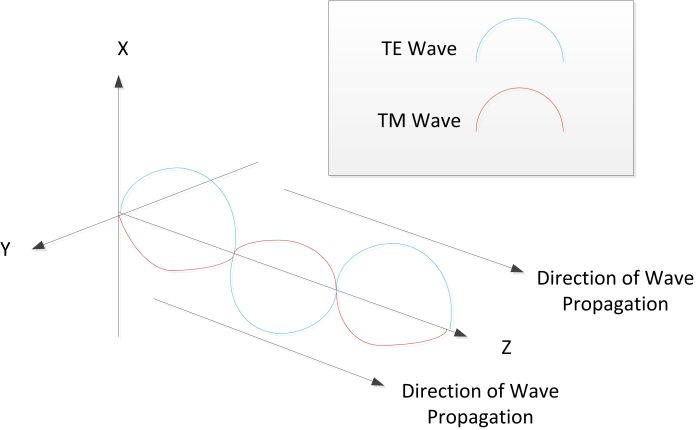Figure 18: Visual Illustration of TE and TM waves

##### Appendix D: Additional Factors to consider

Some refraction will happen at the LED which could affect the resultant estimation of the receiver's position.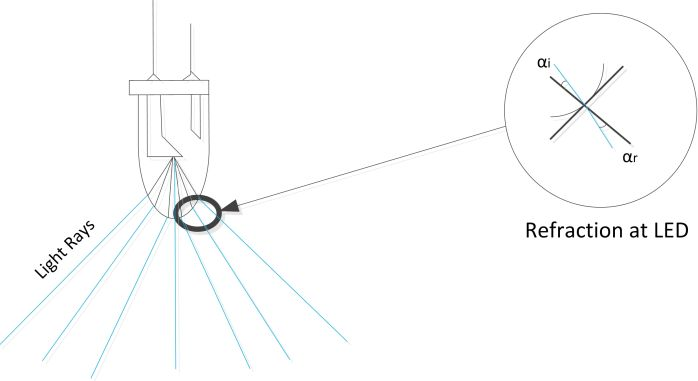Figure 19: Additional Issue: Light rays refracting at LED

Inevitably, there will also be some colour dispersions (Pedrotti L. F. et al., 2007) once the light ray refracts through the hemispherical lens which could result in a miscalculation of the location of brightest pixel, and consequently, a wrong estimate of the receiver's position (Figure 20).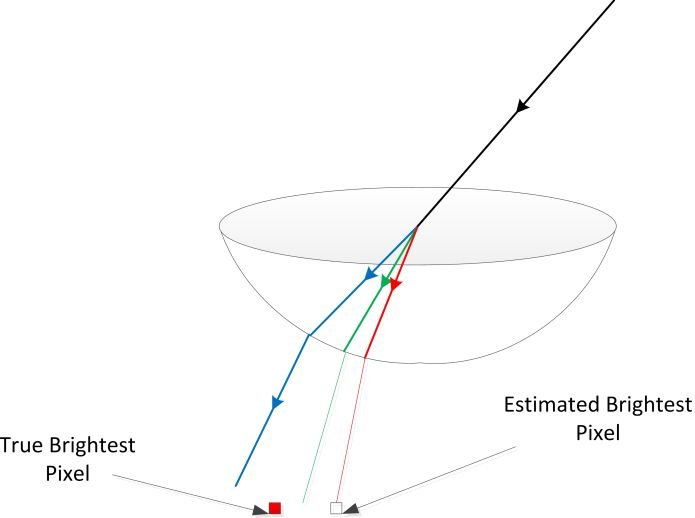Figure 20: Additional issue: Colour Dispersion

Furthermore, misalignment between the hemispherical lens and the grid is another issue which could also misinterpret the location of the brightest pixel.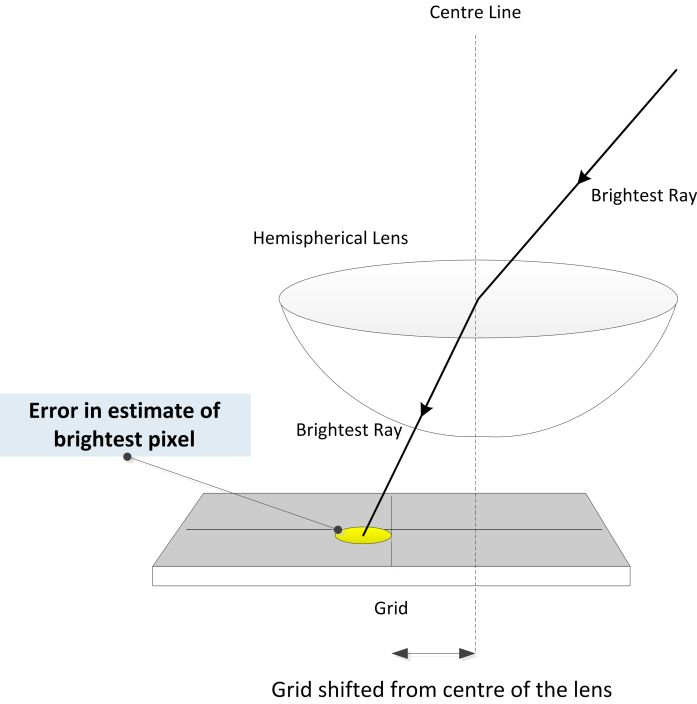Figure 21: Additional issue: Misalignment between grid and lens

## Notes

 Jiun Bin Choong is in his penultimate year, studying for Bachelor of Engineering and Bachelor of Commerce at Monash University in Melbourne, Australia. Recently, He was awarded for "Best spoken presentation" under his division at the International Conference of Undergraduate Research held by the Monash Warwick Alliance. He would like to continue research once he has completed his double degree course.

## References

Grubor, J., S. Randel, L. Klaus-Dieter and J. W. Walewski (2008), 'Broadband Information Broadcasting Using LED-Based Interior Lighting', Lightwave Technology, Journal of 26 (24), 3883-92

Guoqiang, M. and F. Baris (2009), Localization algorithms and strategies for wireless sensor networks, Hershey, Pa.: Information Science Reference

Hallert, B. (1960), Photogrammetry, York, Pa.: The Maple Press Company

Hui, L., H. Darabi, P. Banerjee and J. Liu (2007), 'Survey of Wireless Indoor Positioning Techniques and Systems', Systems, Man, and Cybernetics, Part C: Applications and Reviews, IEEE Transactions on, 37 (6), 1067-80

Kahn, J. M. and J. R. Barry (1997), 'Wireless infrared communication', Proceedings of the IEEE, 85 (2), 265-98

Mautz, R. and S. Tilch (2011), 'Survey of optical indoor positioning systems', Indoor Positioning and Indoor Navigation (IPIN), 2011 International Conference

Pedrotti L. F., L. S. Pedrotti and L. M. Pedrotti (2007), Introduction to optics, Upper Saddle River, NJ.: Pearson Prentice Hall

Rahman, M. S., M. M. Haque and K. Ki-Doo (2011), 'High precision indoor positioning using lighting LED and image sensor', Computer and Information Technology (ICCIT), 2011 International Conference

Swook, H., K. Jung-Hun, J. Soo-Young and P. Chang-Soo (2010), 'White LED ceiling lights positioning systems for optical wireless indoor applications', Optical Communication (ECOC), 2010 European Conference and Exhibition

Uchiyama, H., M. Yoshino, H. Saito, M. Nakagawa, S. Haruyama, T. Kakehashi and N. Nagamoto (2008), 'Photogrammetric system using visible light communication', Industrial Electronics, IECON 2008, 34th Annual Conference of the IEEE

Wang, T. Q., Y. A. Sekercioglu and J. Armstrong (2013), 'Analysis of An Optical Wireless Receiver Using A Hemispherical Lens with Application in MIMO Visible Light Communications', Journal of Lightwave Technology, 31 (11), 1744-54

To cite this paper please use the following details: Choong, J. (2013), 'Future indoor Global Positioning System (GPS) using white LEDs?', Reinvention: an International Journal of Undergraduate Research, BCUR/ICUR 2013 Special Issue, href="http://www.warwick.ac.uk/reinventionjournal/issues/bcur2013specialissue/choong/. Date accessed [insert date]. If you cite this article or use it in any teaching or other related activities please let us know by e-mailing us at Reinventionjournal at warwick dot ac dot uk.